# SE 09 (test design techs).pptx

May. 27, 2023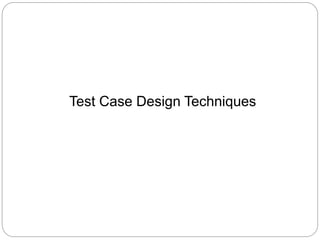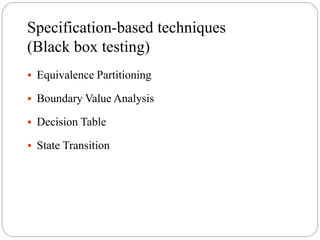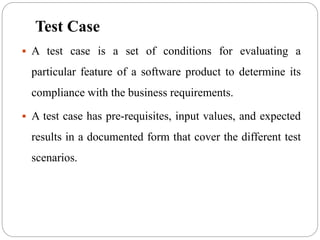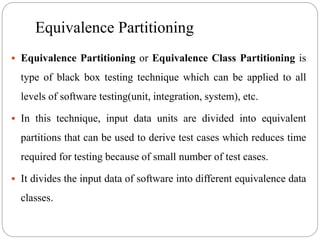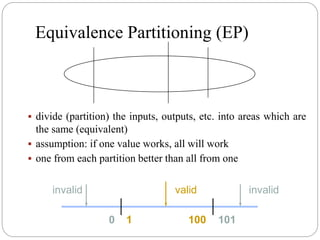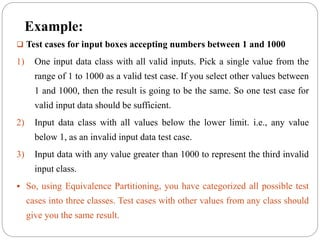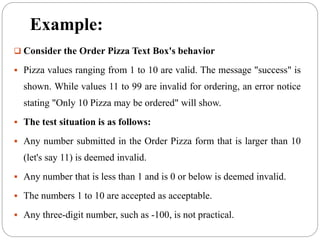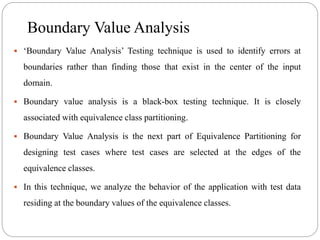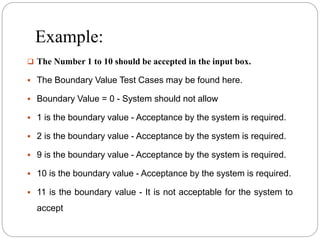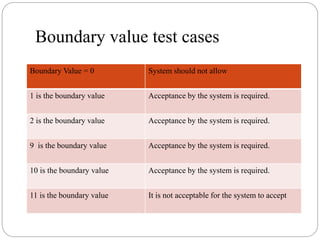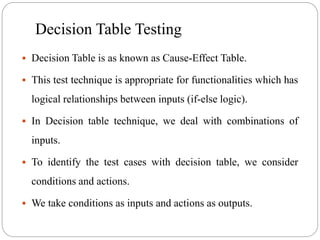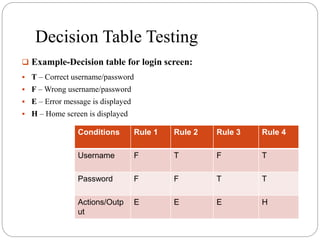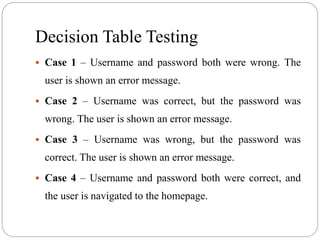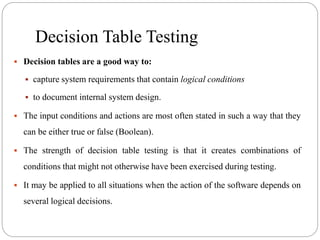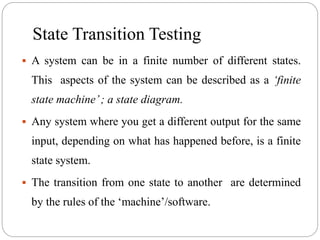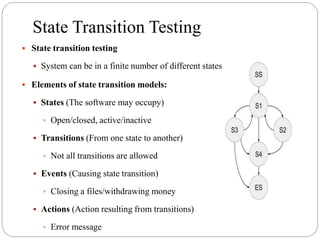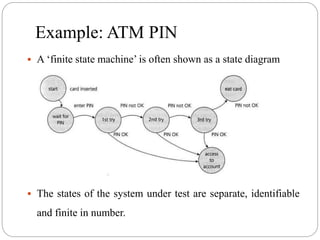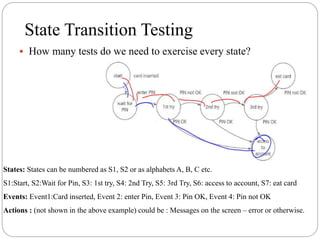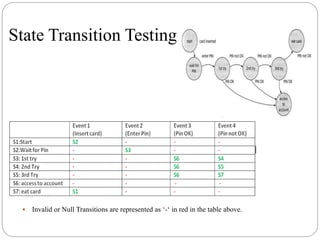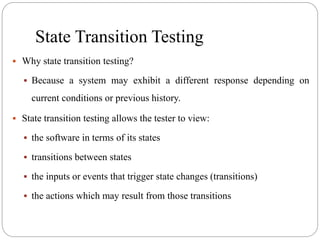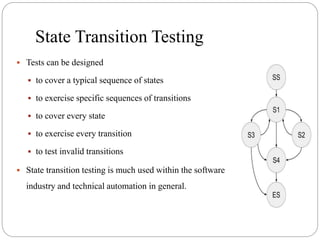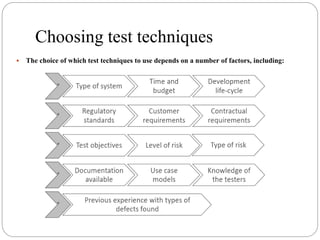1 of 23

### SE 09 (test design techs).pptx

• 2. Test Case Design Techniques
• 3. Specification-based techniques (Black box testing)  Equivalence Partitioning  Boundary Value Analysis  Decision Table  State Transition
• 4. Test Case  A test case is a set of conditions for evaluating a particular feature of a software product to determine its compliance with the business requirements.  A test case has pre-requisites, input values, and expected results in a documented form that cover the different test scenarios.
• 5. Equivalence Partitioning  Equivalence Partitioning or Equivalence Class Partitioning is type of black box testing technique which can be applied to all levels of software testing(unit, integration, system), etc.  In this technique, input data units are divided into equivalent partitions that can be used to derive test cases which reduces time required for testing because of small number of test cases.  It divides the input data of software into different equivalence data classes.
• 6. Equivalence Partitioning (EP)  divide (partition) the inputs, outputs, etc. into areas which are the same (equivalent)  assumption: if one value works, all will work  one from each partition better than all from one 1 100 101 0 valid invalid invalid
• 7. Example:  Test cases for input boxes accepting numbers between 1 and 1000 1) One input data class with all valid inputs. Pick a single value from the range of 1 to 1000 as a valid test case. If you select other values between 1 and 1000, then the result is going to be the same. So one test case for valid input data should be sufficient. 2) Input data class with all values below the lower limit. i.e., any value below 1, as an invalid input data test case. 3) Input data with any value greater than 1000 to represent the third invalid input class.  So, using Equivalence Partitioning, you have categorized all possible test cases into three classes. Test cases with other values from any class should give you the same result.
• 8. Example:  Consider the Order Pizza Text Box's behavior  Pizza values ranging from 1 to 10 are valid. The message "success" is shown. While values 11 to 99 are invalid for ordering, an error notice stating "Only 10 Pizza may be ordered" will show.  The test situation is as follows:  Any number submitted in the Order Pizza form that is larger than 10 (let's say 11) is deemed invalid.  Any number that is less than 1 and is 0 or below is deemed invalid.  The numbers 1 to 10 are accepted as acceptable.  Any three-digit number, such as -100, is not practical.
• 9. Boundary Value Analysis  ‘Boundary Value Analysis’ Testing technique is used to identify errors at boundaries rather than finding those that exist in the center of the input domain.  Boundary value analysis is a black-box testing technique. It is closely associated with equivalence class partitioning.  Boundary Value Analysis is the next part of Equivalence Partitioning for designing test cases where test cases are selected at the edges of the equivalence classes.  In this technique, we analyze the behavior of the application with test data residing at the boundary values of the equivalence classes.
• 10. Example:  The Number 1 to 10 should be accepted in the input box.  The Boundary Value Test Cases may be found here.  Boundary Value = 0 - System should not allow  1 is the boundary value - Acceptance by the system is required.  2 is the boundary value - Acceptance by the system is required.  9 is the boundary value - Acceptance by the system is required.  10 is the boundary value - Acceptance by the system is required.  11 is the boundary value - It is not acceptable for the system to accept
• 11. Boundary value test cases Boundary Value = 0 System should not allow 1 is the boundary value Acceptance by the system is required. 2 is the boundary value Acceptance by the system is required. 9 is the boundary value Acceptance by the system is required. 10 is the boundary value Acceptance by the system is required. 11 is the boundary value It is not acceptable for the system to accept
• 12. Decision Table Testing  Decision Table is as known as Cause-Effect Table.  This test technique is appropriate for functionalities which has logical relationships between inputs (if-else logic).  In Decision table technique, we deal with combinations of inputs.  To identify the test cases with decision table, we consider conditions and actions.  We take conditions as inputs and actions as outputs.
• 13. Decision Table Testing  Example-Decision table for login screen:  T – Correct username/password  F – Wrong username/password  E – Error message is displayed  H – Home screen is displayed Conditions Rule 1 Rule 2 Rule 3 Rule 4 Username F T F T Password F F T T Actions/Outp ut E E E H
• 14. Decision Table Testing  Case 1 – Username and password both were wrong. The user is shown an error message.  Case 2 – Username was correct, but the password was wrong. The user is shown an error message.  Case 3 – Username was wrong, but the password was correct. The user is shown an error message.  Case 4 – Username and password both were correct, and the user is navigated to the homepage.
• 15. Decision Table Testing  Decision tables are a good way to:  capture system requirements that contain logical conditions  to document internal system design.  The input conditions and actions are most often stated in such a way that they can be either true or false (Boolean).  The strength of decision table testing is that it creates combinations of conditions that might not otherwise have been exercised during testing.  It may be applied to all situations when the action of the software depends on several logical decisions.
• 16. State Transition Testing  A system can be in a finite number of different states. This aspects of the system can be described as a ‘finite state machine’; a state diagram.  Any system where you get a different output for the same input, depending on what has happened before, is a finite state system.  The transition from one state to another are determined by the rules of the ‘machine’/software.
• 17. State Transition Testing  State transition testing  System can be in a finite number of different states  Elements of state transition models:  States (The software may occupy)  Open/closed, active/inactive  Transitions (From one state to another)  Not all transitions are allowed  Events (Causing state transition)  Closing a files/withdrawing money  Actions (Action resulting from transitions)  Error message
• 18. Example: ATM PIN  A ‘finite state machine’ is often shown as a state diagram  The states of the system under test are separate, identifiable and finite in number.
• 19. State Transition Testing  How many tests do we need to exercise every state? States: States can be numbered as S1, S2 or as alphabets A, B, C etc. S1:Start, S2:Wait for Pin, S3: 1st try, S4: 2nd Try, S5: 3rd Try, S6: access to account, S7: eat card Events: Event1:Card inserted, Event 2: enter Pin, Event 3: Pin OK, Event 4: Pin not OK Actions : (not shown in the above example) could be : Messages on the screen – error or otherwise.
• 20. State Transition Testing  Invalid or Null Transitions are represented as ‘-‘ in red in the table above.
• 21. State Transition Testing  Why state transition testing?  Because a system may exhibit a different response depending on current conditions or previous history.  State transition testing allows the tester to view:  the software in terms of its states  transitions between states  the inputs or events that trigger state changes (transitions)  the actions which may result from those transitions
• 22. State Transition Testing  Tests can be designed  to cover a typical sequence of states  to exercise specific sequences of transitions  to cover every state  to exercise every transition  to test invalid transitions  State transition testing is much used within the software industry and technical automation in general.
• 23. Choosing test techniques  The choice of which test techniques to use depends on a number of factors, including: